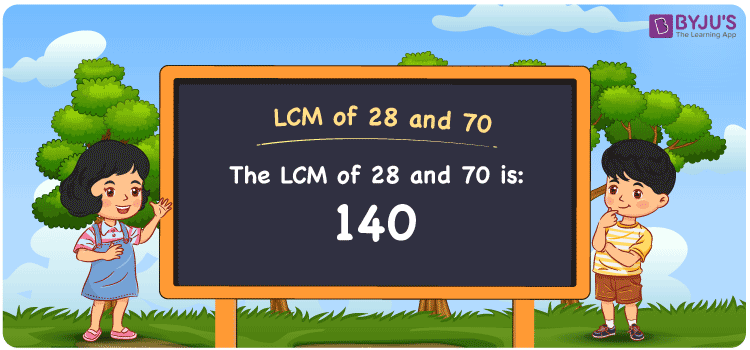# LCM of 28 and 70

LCM of 28 and 70 is 140. LCM stands for the Least Common multiple or Lowest Common Multiple. LCM is the smallest number that is divisible by the given numbers. In the given set of numbers 28 and 70, 140 is the first(least or smallest) number that is common in the set of common multiples of 28 and 70. The LCM which is also known as LCD, Least Common Divisor for 28 and 70 is 140. You can read more on LCM for further details.

## What is LCM of 28 and 70

The Least Common Multiple or Lowest Common Multiple of 28 and 70 is 140.## How to Find LCM of 28 and 70?

LCM of 28 and 70 can be determined using three methods:

• Prime Factorisation
• Division method
• Listing the multiples

### LCM of 28 and 70 Using Prime Factorisation Method

In the Prime Factorisation method, the numbers 28 and 70 can be expressed as;

28 = 2 × 2 × 7

70 = 2 × 5 × 7

2 × 7 is the common factor for 28 and 70. The other factors of these two numbers are multiplied with 2 × 7 to get the LCM.

LCM (28, 70) = 2 × 2 × 5 × 7 = 140

### LCM of 28 and 70 Using Division Method

In the Division Method, the numbers 28 and 70 are divided together with common prime divisors. The division is completed with other prime divisors also till the remainder becomes one. The product of the prime divisors forms the LCM.

 2 28 70 2 14 35 5 7 35 7 7 7 × 1 1

Therefore LCM (28, 70) = 2 × 2 × 5 × 7 = 140

### LCM of 28 and 70 Using Listing the Multiples

By listing all the multiples of 28 and 70, we can identify the LCM. Below is the list of multiples for 28 and 70

 Multiples of 28 Multiples of 70 28 70 56 140 84 210 112 280 140 350

LCM (28, 70) = 140

## Video Lesson on Applications of LCM## Solved Examples

What is the smallest number that is divisible by both 28 and 70?

Answer: 140 is the smallest number that is divisible by both 28 and 70.

What is the LCM for 7, 28 and 70?

Answer: LCM for 7, 28 and 70 is 140 as 7 is the factor of 28 and 70.

## Frequently Asked Questions on LCM of 28 and 70

### What is the LCM of 28 and 70?

The LCM of 28 and 70 is 140.

### Is the LCM of 28 and 70 the same as the Highest Common Factor of 28 and 70?

No. The Least Common Multiple of 28 and 70 is 140 and the Highest Common Factor of 28 and 70 is 14.

### What are the common factors of 28 and 70?

1, 2, 7 and 14 are the common factors of 28 and 70.

### What are the first 3 common multiples of 28 and 70?

The first three common multiples of 28 and 70 are 140, 280, and 420.

### Is the LCM of 7 and 28 same as the LCM of 7 and 70?

No. LCM of 7 and 28 is 28, whereas the LCM of 7 and 70 is 70. They are not the same.